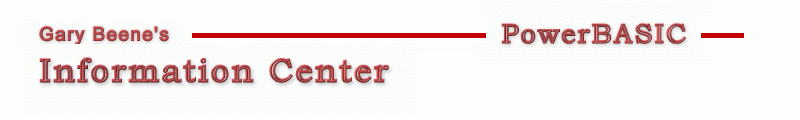## Equate Check

Category: Source Code Analysis

Date: 03-28-2012

'Sometimes I add a lot of equates to a program, then make major changes to the
'code and no longer need all of the equates.  This utility detects which equates
'are no longer used.

'Primary Code:
'The function EquateCheck below does all the work. For length reasons, the code
'is only shown below, but here's a list of the steps it performs:

'1. Remove all unallowed characters (all except 0-9, a-z, A-Z, %, and _ )
'2. Put all equates in a sorted array
'3. Use TALLY to see if equates only 1 time in the test code

'The code does not test whether an equates is found within a comment, but
'in my experience that rarely happens so the code is simpli

'Compilable Example:
#Compiler PBWin 9, PBWin 10
#Compile EXE
#Dim All
#Include "Win32API.inc"
Global hDlg as DWord

Function PBMain() As Long
Local style&
style& = %ws_tabstop Or %ws_border Or  %es_left Or %es_autohscroll _
Or %es_multiline Or %es_nohidesel Or %es_wantreturn
Dialog New Pixels, 0, "Test Code",300,300,250,350, %WS_OverlappedWindow To hDlg
Control Add Button, hDlg, 100,"Push", 10,10,100,20
Dialog Show Modal hDlg Call DlgProc
End Function

CallBack Function DlgProc() As Long
If CB.Msg = %WM_Command AND CB.Ctl = 100 Then
Local temp\$
Control Get Text hDlg, 200 To temp\$
EquateCheck temp\$
End If
End Function

Local temp\$
temp\$ = "#Compile EXE"
temp\$ = temp\$ + \$crlf + "#Dim All"
temp\$ = temp\$ + \$crlf + "%ID_Flag = 500"
temp\$ = temp\$ + \$crlf + "%ID_Marker = 600"
temp\$ = temp\$ + \$crlf + "%ID_Btn = 700"
temp\$ = temp\$ + \$crlf + "Function PBMain() As Long"
temp\$ = temp\$ + \$crlf + "   Local i,j As Long
temp\$ = temp\$ + \$crlf + "   i = %ID_Flag
temp\$ = temp\$ + \$crlf + "   j = %ID_Marker
temp\$ = temp\$ + \$crlf + "End Function"
Function = temp\$
End Function

Sub EquateCheck(temp\$)
Local UnusedEquates\$, i, iPos As Long

'Remove all unallowed chars (replace with \$spc)
Replace ":" With \$CrLf In temp\$
For i = 0 To 127  '255 would be safer
Select Case i
Case 48 To 57, 65 To 90, 97 To 122, 37, 95, 10, 13  'no action if 0-9, A-Z, a-z, %, _
Case Else : Replace Chr\$(i) With \$Spc In temp\$      'remove all chars not part of equate names
End Select
Next i

'put all lines in an array
Dim Equates() As String
ReDim Equates(ParseCount(temp\$,\$CrLf)-1)
Parse temp\$, Equates(), \$CrLf

'create a sorted array of all lines (trim\$'d)
'any line which does not DEFINE an equate is set to \$nul
For i = 0 To UBound(Equates)
Equates(i) = LTrim\$(Equates(i)) + \$Spc  'make sure there is a following \$spc after every equate
If Left\$(Equates(i),1) = "%Then
iPos = Instr(Equates(i),\$Spc)
Equates(i) = Left\$(Equates(i),iPos)  'line now contains equate + \$spc
End If
Next i
Array Sort Equates(), Ascend

'create list of unused equate (unused means appers only 1 time in temp\$)
Replace \$CrLf With \$Spc In temp\$    'ensures a trailing \$spc when an equate is by itself on a line
For i = 0 To UBound(Equates)
If Left\$(Equates(i),1) = "%Then
If Tally(temp\$, Equates(i)) = 1 Then
UnusedEquates\$ = UnusedEquates\$ + Equates(i)
Replace Equates(i) With " " In temp\$
End If
End If
Next i

? "Unused Equates: " + IIF\$(Len(UnusedEquates), UnusedEquates, "None")

End Sub

'gbs_00543
'Date: 03-10-2012

created by gbSnippets
http://www.garybeene.com/sw/gbsnippets.htm